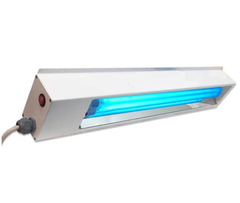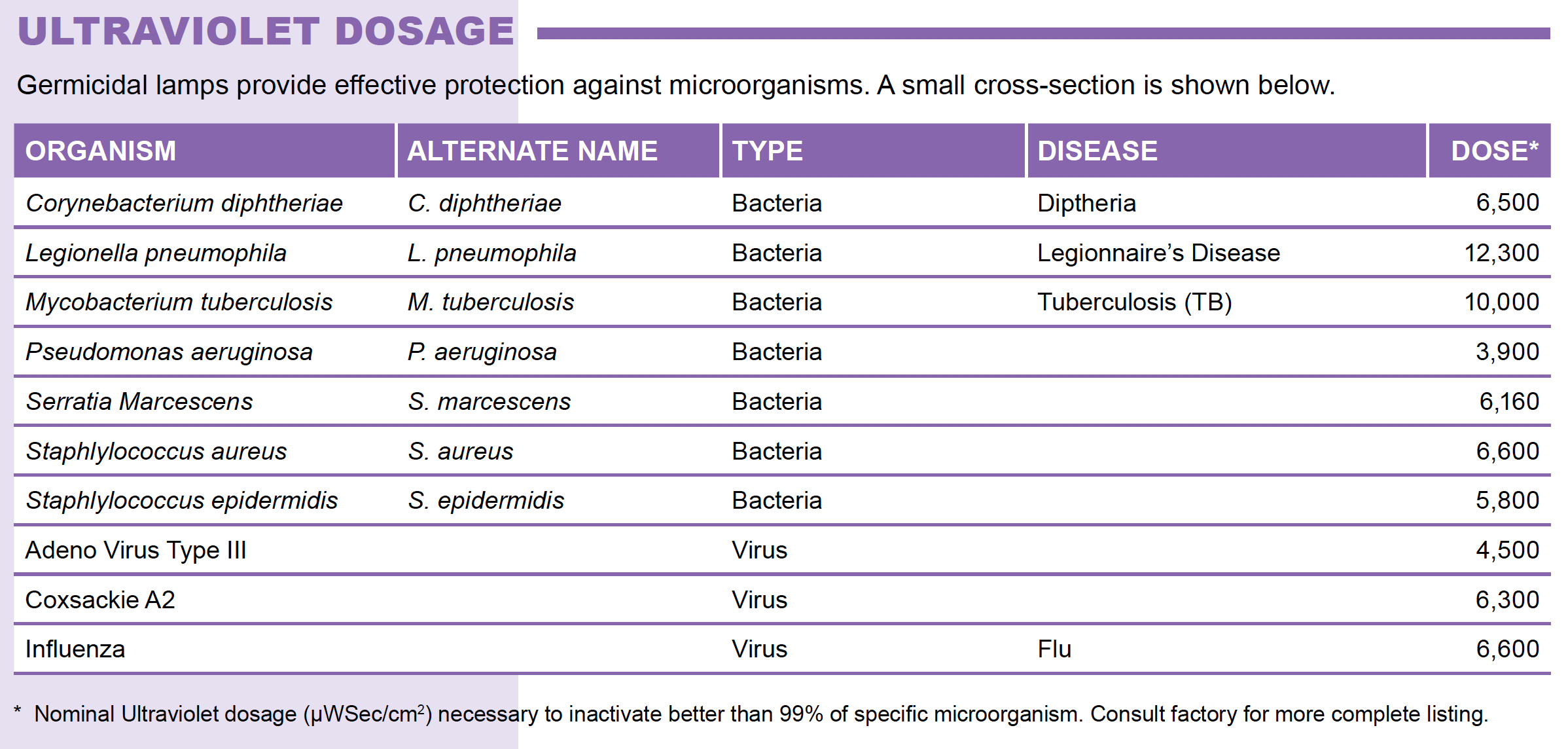# How to Calculate UV-C Dose on a Surface

Posted by on for ProLampSalesWhen UV-C fixtures or handheld devices are used to sanitize surfaces and objects, it may be useful to determine the UV dose applied and comparing that to tables of dose requirements for deactivating microorganisms.

## Measure UV Intensity

To get accurate dose data you need to start with a UV-C detector. The detector needs to display an intensity reading measured in microwatts per square centimeter (μW/cm² ). The Zenith detector is one example of a UV-C detector that will work well for this purpose.

With the UV-C fixture or handheld device turned on, the detector should be placed as close as possible to the same distance away from the UV-C source (lamp) as when it is being used to sanitize surfaces or objects. The meter will display the UV intensity in μW/cm².

## Calculate UV Dose

UV Dose = UV Intensity (μW/cm² ) x Exposure Time (seconds)

Exposure time is how long the UV light is ON and delivering UV to a surface. Start with 20 or 30 seconds.

The result of this calculation will be a value expressed in  μWSec/cm².

## Compare Dose Calculation to Dose Required to Disable Various Pathogens

This value can be compared to the table below for various microorganisms. To deactivate any of these organisms, the value should match or exceed the dose values shown. You can simply change the Exposure Time until you reach the dose value you need.• @Mark, could you tell me how you come up with the formulas?

Nico on
• @Carmelo
What are your bulbs rated for? Check your bulb spec sheet. It should show a value for Radiated Power. That is the ‘real’ wattage you should be using in your calculations.

Bully on
• How about Distance? If I have a 4 footer dual tube 30W UVC bulb on a 80 feet ceiling, how long will it need to be on? And how long would it cover?

Carmelo Milian on
• @Mark

Thank you so much for these formulas! Your calculations work out but I do have one question.
My bulbs are rated at 18 watts. 18/ (2 π×2.54×102) This returns a value of 0.01105754583. In your example of .9 watts at 2.54cm. .9/ (2 π×2.54×8.5) returns a value of 0.0066345275.
Your final answer is 6.64 mW/cm2. Why are we using the 5th place value? Thanks for any advice.

Bully on
• Sorry had few missing words from my final example… so this is a correction to my last post….

A) For a large distance to the lamp we get:
The irradiance = (Total Radiation flux (W)/ π2.a2 ——- For (a >> L)

B) At shorter distances the irradiance:
The irradiance = (Total Radiation flux (W)/ 2 π.a.L ——- For (a < 0.5 L)

Where total Radiation flux for 4W bulb is 0.9 w as you indicated
L: is arc length of the lamb which is distance between 2 arcs inside lamb tube which is 3.35” (85mm) for 4 Watt lamb
a: Is the perpendicular distance between surface & Bulb

If we assume distance is 1 meter as you stated then if you run through the calculation using A) formula >>>>> the irradiance would equal to 9.13 uW/cm2

If you want to calculate irradiance at 1 inch (2.54cm) then use formula B (since distance is smaller than half of the bulb length) >>>>> the irradiance would equal to 6.64 mW/cm2

Finally for distance of 4” (10.16cm) >>>>> the irradiance would equal to 884 uW/cm2

Using above 2 equations you could calculate irradiance at any distance.

Don’t forget these bibs operate at 254nm wavelength, and peak germicidal effect occurs at 260 to 264nm so you need to correct for the radiation generated by your bulb at 254 nm, which is approximately at 85% of the maximum germicidal effect. So derate you’re calculated irradiance by 15%. Hope this helps.
Mark Qandil

Mark Qandil on